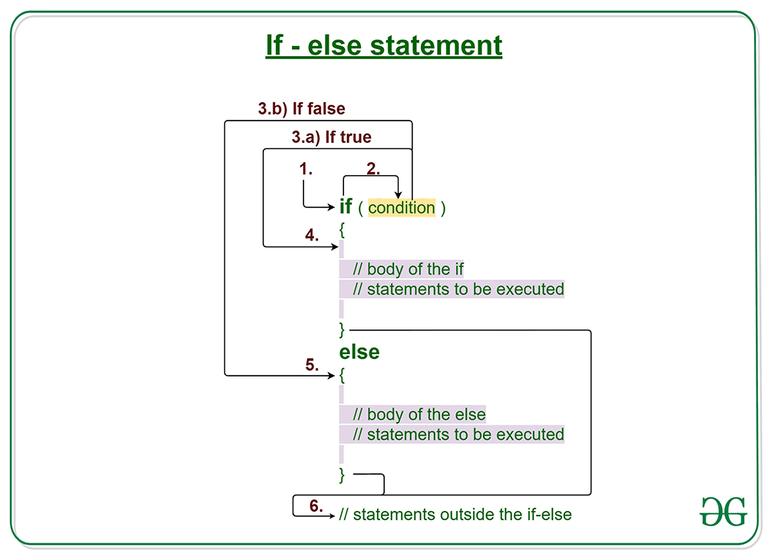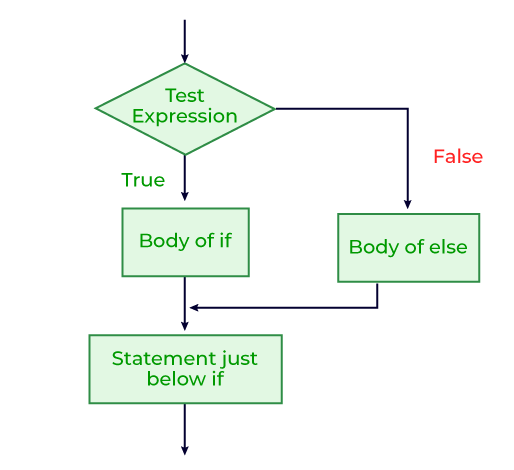GFG App
Open AppBrowser
Continue

Decision Making in Java helps to write decision-driven statements and execute a particular set of code based on certain conditions. The if statement alone tells us that if a condition is true it will execute a block of statements and if the condition is false it won’t. But what if we want to do something else if the condition is false. Here comes the else statement.

We can use the else statement with the if statement to execute a block of code when the condition is false. If- else together represents the set of Conditional statements in Java that are executed according to the condition which is true.## Syntax of if else statement

```if (condition)
{
// Executes this block if
// condition is true
}
else
{
// Executes this block if
// condition is false
}```

## Working on if-else statements in Java

If-else statements are one of the most efficient ways to use conditional statements and to get the benefit out of them. Below is the working if-else statements in Java:

1. Control falls into the if block.
2. The flow jumps to Condition.
3. Condition is tested.
• If Condition yields true, go to Step 4.
• If Condition yields false, go to Step 5.
4. The if-block or the body inside the if is executed.
5. The else block or the body inside the else is executed.
6. Flow exits the if-else block.

## If-else flowchart## Java if-else examples

### Example 1

#### Dry-Run of if-else statements

```1. Program starts.
2. i is initialized to 20.
3. if-condition is checked. 20<15, yields false.
4. flow enters the else block.
4.a) "i is greater than 15" is printed
5. "Outside if-else block" is printed.```

## Java

 `// Java program to illustrate if-else statement ` ` `  `class` `IfElseDemo { ` `    ``public` `static` `void` `main(String args[]) ` `    ``{ ` `        ``int` `i = ``20``; ` ` `  `        ``if` `(i < ``15``) ` `            ``System.out.println(``"i is smaller than 15"``); ` `        ``else` `            ``System.out.println(``"i is greater than 15"``); ` ` `  `        ``System.out.println(``"Outside if-else block"``); ` `    ``} ` `}`

Output

```i is greater than 15
Outside if-else block```

### Example 2

The first print statement is in a block of “if” so the second statement is not in the block of “if”. The third print statement is in else but that else doesn’t have any corresponding “if”. That means an “else” statement cannot exist without an “if” statement.

Below is the implementation of the above approach:

## Java

 `// Java program to illustrate if-else statement ` ` `  `class` `IfElseDemo { ` `    ``public` `static` `void` `main(String args[]) ` `    ``{ ` `        ``int` `x=``10``; ` ` `  `        ``if` `(x == ``10``) ` `            ``System.out.println(``"Hello geek"``); ` `              ``System.out.println(``"Hi geek"``); ` `        ``else` `            ``System.out.println(``"Welcome to GeeksforGeeks"``); ` `    ``} ` `}`

```./IfElseDemo.java:10: error: 'else' without 'if'
else
^
1 error```

### 1. What is the if-else in Java?

If-else is conditional statements that execute according to the correct statement executed. If the if condition is true then the code block inside the if statement is executed else it executes the else statement block.

### 2. What is the syntax for if-else?

The syntax for if-else:

```if (condition)
{
// Executes this block if
// condition is true
}
else
{
// Executes this block if
// condition is false
}```

## Related Topics

My Personal Notes arrow_drop_up